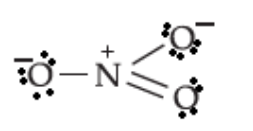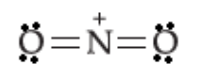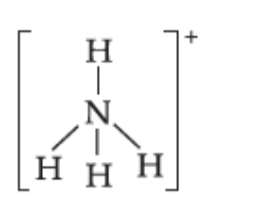QuestionAnswers

# The hybridisation of the orbitals of N atom in ${\rm{NO}}_3^ - {\rm{, NO}}_2^ +$ and ${\rm{NH}}_4^ +$ are respectively:A) $sp,s{p^2},s{p^3}$B) $s{p^2},sp,s{p^3}$ C) $sp,s{p^3},s{p^2}a$ D) $s{p^2},sp,sp$

Hint: Determine the number of atoms that are joined to the central atom. Also take into account the lone pairs to determine the hybridization.

Write the lewis dot structure of ${\rm{NO}}_3^ -$:The central nitrogen atom has three bonding domains and zero lone pairs of electrons. Three bonding domains are obtained when three hybrid orbitals of nitrogen overlap with orbitals of three oxygen atoms. Three hybrid orbitals on nitrogen atom will be obtained by the hybridisation of three atomic orbitals of nitrogen atom. Thus, the nitrogen atom is $s{p^2}$ hybridised. The geometry of the ion is trigonal planar.

Write the lewis dot structure of ${\rm{NO}}_2^ +$:The central nitrogen atom has two bonding domains and zero lone pairs of electrons. Two bonding domains are obtained when two hybrid orbitals of nitrogen overlap with orbitals of two oxygen atoms. Two hybrid orbitals on nitrogen atom will be obtained by the hybridisation of two atomic orbitals of nitrogen atom. Thus, the nitrogen atom is $sp$ hybridised. The geometry of the ion is linear.

Write the lewis dot structure o ${\rm{NH}}_4^ +$:The central nitrogen atom has four bonding domains and zero lone pairs of electrons. Four bonding domains are obtained when four hybrid orbitals of nitrogen overlap with orbitals of four oxygen atoms. Four hybrid orbitals on nitrogen atom will be obtained by the hybridisation of four atomic orbitals of nitrogen atom. Thus, the nitrogen atom is $s{p^3}$ hybridised. The geometry of the ion is tetrahedral.

Hence, the hybridisation of the orbitals of N atom in ${\rm{NO}}_3^ - {\rm{, NO}}_2^ +$ and ${\rm{NH}}_4^ +$ are $s{p^2},sp,s{p^3}$ respectively.

Hence, option B) is the correct answer.

Note:
When a double bond is present, do not count it as two bonding domains. Consider it as only one bonding domain.